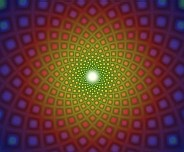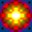Fibonacci

# Fibonacci SpiralsThe NatIntelCustom Fibonacci Spiral Generator You can make your own Fibonacci spirals with thisnew application.  Also, a demo is now available.

### New Fibonacci Images

I call this type of design a "Fibonacci spiral" because the math used for it can be related to a Fibonacci sequence. An example of a Fibonacci sequence is 1,1,2,3,5,8,13,21,34,55,... where each term after the second one is the sum of the two preceding terms. The ratio of each term to the previous one gradually converges to a limit of approximately 1.618 (which, incidentally, is also the Golden Mean). The designs on this page were created with a series of Visual Basic programs.

#### Fixed Ratio in Spirals

At each concentric layer of a Fibonacci spiral, the ratio of a single component piece to one from the next inner layer is a constant, which varies according to how many radially symmetric parts of the spiral there are. This makes the expanding sequence of layers a kind of Fibonacci sequence: each one is a certain linear combination of previous ones.

These spirals occur frequently in nature, for example in a Nautilus shell or the center of a sunflower. It seems that each growing layer of the organism must reproduce itself in a certain expanded version one layer out, thus guaranteeing a Fibonacci pattern.

The individual components for the spirals were designed using a special version of the Symmetry program, and then run through a color progression as they move outwards.

Fibonacci Spirals

Last Updated July 11th, 2004
Web Page by Ned May
URL http://chromatism.net/chromat/fibonaci.htm